Definitions of Square Dance Calls and Concepts
Locker's Choice [C3A]

Index -->  Plus  |  A1  |  A2  |  C1  |  C2  |  C3A  |  C3B  |  C4  |  NOL  |
Definitions (Text Only) -->  Plus  |  A1  |  A2  |  C1  |  C2  |  C3A  |  C3B  |  C4  |  NOL  |
 Find call:

 \$B8@8l(B\$BJ?9T\$J(B Wave \$B\$+\$i!%(B

1. Lockit;
2. Center Wave \$B\$O(B Lockit \$B\$r(B, \$BB>\$N?M\$O(B Trade \$B\$r\$7(B,
3. Center Wave \$B\$O(B Hinge \$B\$r(B, \$BB>\$N?M\$O(B Step & Fold \$B\$r\$7\$^\$9!%(B

\$B5U\$N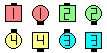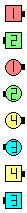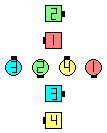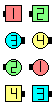Locker's Choice\$B\$NA0(B Lockit(1/3)\$B\$N8e(B Centers Lockit as Outsides Trade(2/3)\$B\$N8e(B Center Wave Hinge as Others Step & Fold\$B\$N8e(B (\$B=*\$o\$j(B)

Choice [C3A]: \$B0lHL2=\$5\$l\$?(B Tidal Line \$B\$^\$?\$OB>\$NE,@Z\$J(B formation \$B\$+\$i!%(BCenter Line|Wave \$B\$O(B Lockit and Hinge \$B\$r(B, \$BB>\$N?M\$O(B Trade and Step & Fold \$B\$r\$7\$^\$9!%(B Tidal Line \$B\$+\$i\$O(B, \$BJ?9T\$J(B Wave \$B\$^\$?\$OJ?9T\$J(B Two-Faced Line \$B\$K\$J\$j\$^\$9!%\$3\$N%3!<%k\$O(B 2 \$B%Q!<%H\$N%3!<%k\$G\$9!%(B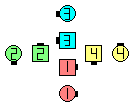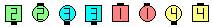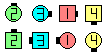Choice\$B\$NA0(B Center Line Lockit as Others Trade\$B\$N8e(B Center Line Hinge as Others Step & Fold\$B\$N8e(B (\$B=*\$o\$j(B)

Cross Locker's Choice [C3B]: Cross Lockit; Choice.CALLERLAB definition for Locker's ChoiceChoreography for Locker's ChoiceComments? Questions? Suggestions?

https://www.ceder.net/def/lockerschoice.php?language=japan&level=C3A
14-December-2019 22:11:23